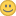# self-tuning cost coefficients

XMLWordPrintable

#### Details

• Type:Task
• Status: Stalled
• Priority:Minor
• Resolution: Unresolved
• Fix Version/s: None
• Component/s: None
• Labels:

#### Description

One of the reasons of bad query plans is inadequate cost estimation of individual operations. A cost of reading a row in one engine might be a lot higher than in some other, but optimizer cannot know it. Also, it uses hard-coded constants, assuming, for example, that evaluating a WHERE clause is 5 times cheaper than reading a row from a table (it used to 10 earlier, now it's 5).

Obviously, some kind of calibration procedure is needed to get these cost estimates to be relatively correct. It is not easy, because the estimates depend on the actual hardware where MariaDB is run (a cost of a row read is different on HD and SSD), and also - somewhat - on the application (optimizer model isn't perfect, and cost factors vary for different types of queries, thus it's better to calibrate on the queries that the user application will run).

A simple and low-maintenance solution would be to use self-tuning cost coefficients. They measure the timing and adjust automatically to the configuration where MariaDB is run.

Assorted thoughts:

• create a tuning coefficient for every low-level cost generator in the optimizer. That is for every function or a handler method that generates a cost value. For every hard-coded constant or a macro too. But not for functions that create cost values from other cost values. For a virtual method - for every implementation of it, that is, one coefficient for every read_time in every handler class. But not for different parameters of it. For example, different tables in some engine might have different costs for reading a row, but we will still have one coefficient for read_time in this engine, not one for each table. The engine is suppose to provide different costs internally, if it needs to. The goal of these coefficients is to normalize the cost between different engines.
• measure the time that the query took, split proportionally between tables (according to the number of rows), save the statistics per coefficient.
• collect the statistics locally in the THD, add to the global statistics on disconnect. it helps to avoid contention on a shared resource
• optimizer will use the global statistics, not thread local. It shouldn't matter, as coefficients will change very slowly.
• in splitting the time use the actual number of rows, not the estimated one, that the optimizer used.
• per coefficient store - counter (bigint), sum of times (double), sum of times squared (double).
• store the results persistently in mysql database
• make them available via the I_S table. In this table show two more columns - the average and the standard deviation.
• report these data via the feedback plugin. we can adjust built-in constants or initial values of these coefficients. and very large deviation is a sign that an engine estimates the cost incorrectly (e.g. doesn't take the table name into account, see above)
• a user can update the table manually, if she wishes so. she can even freeze the coefficients by setting the count column to the very large value.
• system load anomalies may introduce undesired changes in the coefficients. is it a problem? should we implement some countermeasures?

#### People

Assignee:Sergei Golubchik
Reporter:Sergei Golubchik
Votes:
2 Vote for this issue
Watchers:
12 Start watching this issue

#### Dates

Created:
Updated: